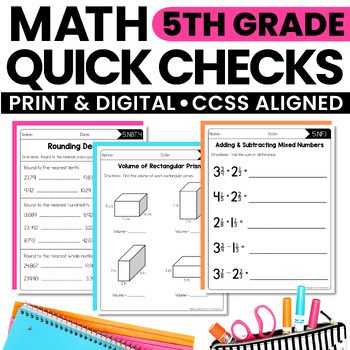# Math Quick Checks - 5th Grade | Math Review Worksheets | Print & Digital3rd - 5th, Homeschool
Subjects
Standards
Resource Type
Formats Included
• PDF
•Google Apps™
Pages
67 pagesThe Teacher-Author indicated this resource includes assets from Google Workspace (e.g. docs, slides, etc.).
##### Also included in
1. What are Interactive Math Notebooks?Interactive math notebooks are teaching pages used to introduce and teach math concepts and skills. Each set of notebook pages includes definitions, explanations, rules, and algorithms that may be needed to address each standard. Practice problems are included to
\$21.50
\$25.75
Save \$4.25
2. This BUNDLE includes everything you need for 5th grade math!!★ Interactive Notebook Pages★ Math Quick Checks ★ Math Menus (Perfect for homework or early finishers!!)★ Open-Ended Math Pages★ Math Exit Slips★ Math Mastery Task Cards★ Printables for DecimalsFeel free to email with any questions or comm
\$57.50
\$70.70
Save \$13.20

### Description

These Math Quick Checks are Common Core aligned. They are exactly what you need to quickly check for understanding or assess student learning. They can be used as assessments, exit tickets, homework, or to help reteach in small groups.

These Quick Checks were originally created to pair with my Interactive Math Notebooks, but alone they are still a great tool to use when teaching Common Core Math Standards.

Includes Digital Pages to use with Google Slides.

Here is a list of titles for each of the worksheets, along with the standard:

Parentheses, Brackets, & Braces (5.OA.1)

Expressions (5.OA.2)

Numerical Patterns, Ordered Pairs, Coordinate Plane (5.OA.3)

Place Value (5.NBT.1)

Powers of 10 (5.NBT.2)

Numerals, Word Form, & Expanded Form (5.NBT.3)

Comparing Decimals (5.NBT.3)

Rounding Decimals (5.NBT.4)

Multiplication (5.NBT.5)

Division (5.NBT.6)

Addition & Subtraction of Decimals (5.NBT.7)

Multiplication of Decimals (5.NBT.7)

Division of Decimals (5.NBT.7)

Adding & Subtracting Mixed Numbers (5.NF.1)

Word Problems: Adding & Subtracting Fractions (5.NF.2)

Relating Fractions to Division (5.NF.3)

Word Problems (5.NF.3)

Multiplying Fractions (5.NF.4)

Area & Fractions (5.NF.4)

Understanding Multiplication of Fractions by Whole Numbers (5.NF.5)

Word Problems: Multiplying Fractions (5.NF.6)

Dividing with Fractions (5.NF.7)

Word Problems: Dividing with Fractions (5.NF.7)

Converting Measurements (5.MD.1)

Word Problems (5.MD.1)

Line Plots (5.MD.2)

Understanding & Measuring Volume (5.MD.3/4)

Volume of Rectangular Prisms (5.MD.5)

Word Problems: Volume (5.MD.5)

Graphing Ordered Pairs (5.G.1/2)

Classifying Shapes (5.G.3/4)

There is an answer key for each page.

Feel free to email me at rebecca@createteachshare.com with any questions or comments.

This product is now included in a BUNDLE!

5th Grade Interactive Math Notebook {BUNDLE}

Check out all of my 5th Grade Interactive Math Notebooks and Worksheets:

5th Grade Interactive Math Notebook: OA & NBT

5th Grade Interactive Math Notebook: NF

5th Grade Interactive Math Notebook: MD & G

Common Core Math Worksheets (for all 5th grade standards)

Total Pages
67 pages
Included
Teaching Duration
1 Year
Report this Resource to TpT
Reported resources will be reviewed by our team. Report this resource to let us know if this resource violates TpT’s content guidelines.

### Standards

to see state-specific standards (only available in the US).
Convert among different-sized standard measurement units within a given measurement system (e.g., convert 5 cm to 0.05 m), and use these conversions in solving multi-step, real world problems.
Make a line plot to display a data set of measurements in fractions of a unit (1/2, 1/4, 1/8). Use operations on fractions for this grade to solve problems involving information presented in line plots. For example, given different measurements of liquid in identical beakers, find the amount of liquid each beaker would contain if the total amount in all the beakers were redistributed equally.
Recognize volume as an attribute of solid figures and understand concepts of volume measurement.
A cube with side length 1 unit, called a “unit cube,” is said to have “one cubic unit” of volume, and can be used to measure volume.
A solid figure which can be packed without gaps or overlaps using 𝘯 unit cubes is said to have a volume of 𝘯 cubic units.